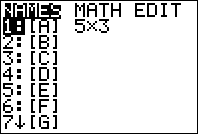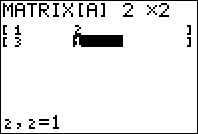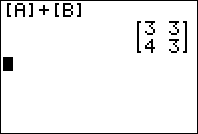# Matrices Made Easy: TI-83 and TI-84 Plus Edition

Filed under Algebra, Difficulty: Easy, TI-83 Plus, TI-84 Plus.

Performing any type of mathematical operation such as addition or multiplication that involves matrices can be a daunting task, especially if you have to do all the steps without the aid of a calculator. Fortunately, your graphing calculator can solve your matrix math problems in seconds, whether you are adding or multiplying matrices or just trying to solve the inverse of a matrix. Keep reading to learn how to work with matrices on your TI-83 or TI-84 graphing calculator.

The first step to working with matrices on your graphing calculator is to specify the dimensions and the values in the matrix.

Starting from your graphing calculator’s home screen, press 2nd . This selects the Matrix menu. You will see a list of letters similar to the screen below:Navigate using the or buttons to select the EDIT option and then press . This allows you to specify the contents of your matrix.

Pressing the or , select the letter corresponding to the matrix you want to use, [A] for this example, then press once more.

For purposes of this example, we’re going to create a simple 2 x 2 matrix. Using the arrows keys to navigate, press followed by another . Now you can enter the values for your matrix. Enter the following sequence of numbers, pressing after inputting each number: 1, 2, 3, 1. Your matrix should look like this following when it is finished:We’re going to go through the same steps again, except instead of selecting Matrix [A], we’re going to select Matrix [B], specifying it as a 2 x 2 matrix, and entering the following sequence of numbers: 2, 1, 1, 2. Press 2nd to return to the home screen once you’re finished.

Now that you have created your matrices, now you can work with them on your graphing calculator. For purposes of this example, we are going to add the two matrices [A] and [B] together.

Press 2nd to select the matrix menu. Instead of going to the EDIT menu like before, we’re simply going to use the or to select [A], then press . This pastes [A] to your home screen. After [A] is on your screen, press the key, then return to the Matrix selection screen and insert [B].
Press and the sum of [A] and [B] will be displayed on your calculator.### More Matrix Fun

Once you have created a matrix on your calculator, you can manipulate and use the matrix in a number of ways. If you want to quickly find the inverse of a matrix, for instance, you can paste it to your home screen following the steps described above, then press the key followed by to find the inverse of the matrix.

Matrix multiplication is extremely easy. Referring to the example above, replace the with a instead and you will solve for the product of the two matrices. Experiment with different matrix combinations and see what you can do with your graphing calculator.